• ## Matlab对图像进行傅里叶变换实例

万次阅读 多人点赞 2019-04-10 22:15:55
使用matlab对peppers.png图像进行二维傅里叶快速变换及逆变换，从简单的实例中发现问题
一、实验目的
1、了解图像变换的意义和手段；
2、熟悉傅里叶变换的基本性质；
3、熟练掌握FFT的方法与应用；
4、通过实验了解二维频谱的分布特点；
5、通过本实验掌握利用MATLAB编程，实现数字图像的傅里叶变换。
二、实验仪器
1、计算机；
2、MATLAB程序；
3、移动式存储器（软盘、U盘等）；
4、记录用的笔、纸。
三、实验原理
1、应用傅里叶变换进行图像处理
傅里叶变换是线性系统分析的一个有力工具，它能够定量地分析诸如数字化系统、采样点、电子放大器、卷积滤波器、噪音和显示点等的作用。通过实验培养这项技能，将有助于解决大多数图像处理问题。对任何想在工作中有效应用数字图像技术的人来说，把时间用在学习和掌握傅里叶变换上是很有必要的。
2、傅里叶（Fourier）变换的定义
对于二维信号，二维Fourier变换定义为：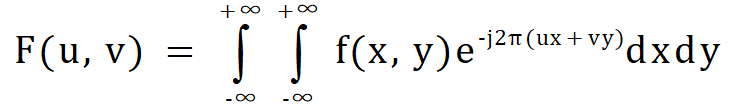逆变换：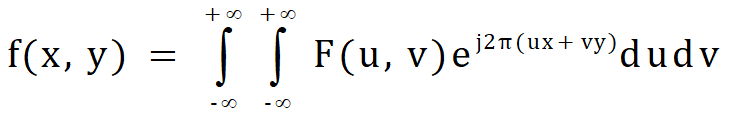二维离散傅里叶变换为：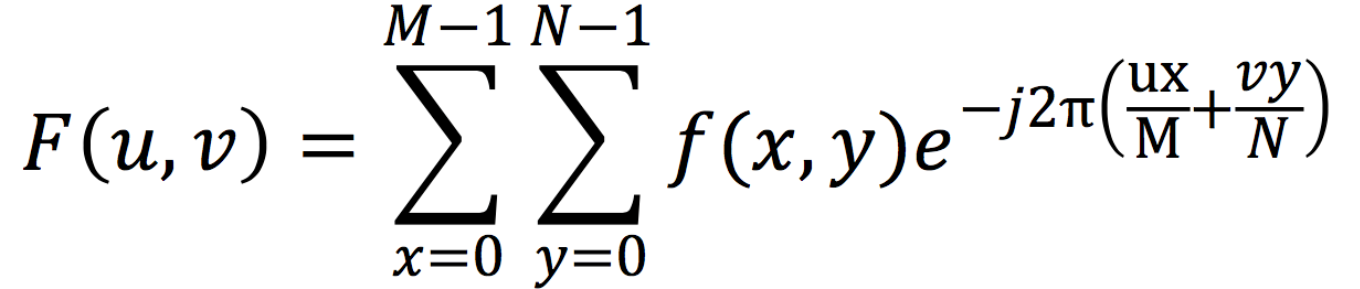逆变换：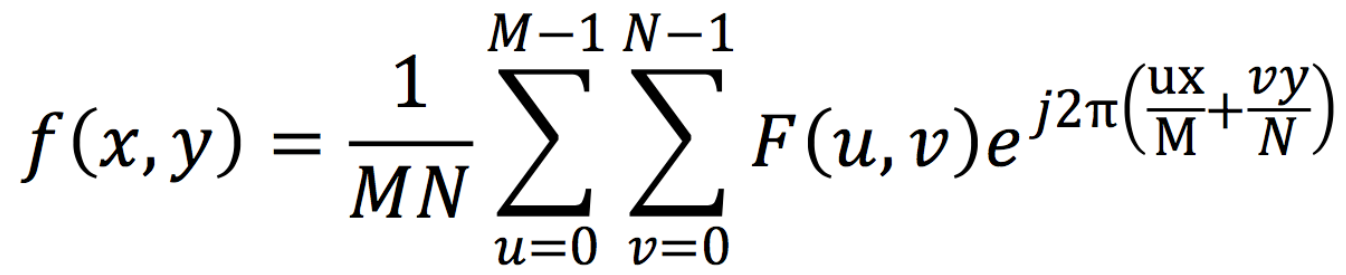图像的傅里叶变换与一维信号的傅里叶变换一样，有快速算法，具体参见参考书目，有关傅里叶变换的快速算法的程序不难找到。实际上，现在有实现傅里叶变换的芯片，可以实时实现傅里叶变换。
3、利用MATLAB软件实现数字图像傅里叶变换、DCT变换的程序。
四、实验步骤
1、打开计算机，安装和启动MATLAB程序；程序组中“work”文件夹中应有待处理的图像文件；
2、利用MATLAB工具箱中的函数绘制FFT频谱显示的函数；
3、a）调入、显示图像；
b）对这图像做FFT、DCT并利用自编的函数显示其频谱；
c）讨论不同的图像内容与FFT、DCT频谱之间的对应关系。
4、记录和整理实验报告。
五、实验内容
MATLAB源程序如下：
clear
clc
subplot(2,2,1);imshow(img);title('原图');
f=rgb2gray(img);    %对于RGB图像必须做的一步，也可以用im2double函数
F=fft2(f);          %傅里叶变换
F1=log(abs(F)+1);   %取模并进行缩放
subplot(2,2,2);imshow(F1,[]);title('傅里叶变换频谱图');
Fs=fftshift(F);      %将频谱图中零频率成分移动至频谱图中心
S=log(abs(Fs)+1);    %取模并进行缩放
subplot(2,2,3);imshow(S,[]);title('频移后的频谱图');
fr=real(ifft2(ifftshift(Fs)));  %频率域反变换到空间域，并取实部
ret=im2uint8(mat2gray(fr));    %更改图像类型
subplot(2,2,4);imshow(ret),title('逆傅里叶变换');

六、实验结果
在MATLAB中运行后，实验结果如图：
在Figure1中，左上角显示的是读入MATLAB程序的原图片，右上角显示的是经过二维傅里叶快速变换后的频谱图像，左下角显示的是将频谱中零频率成分移至矩阵的中心后的频谱图像。右下角显示的是二维傅里叶逆变换后的图像。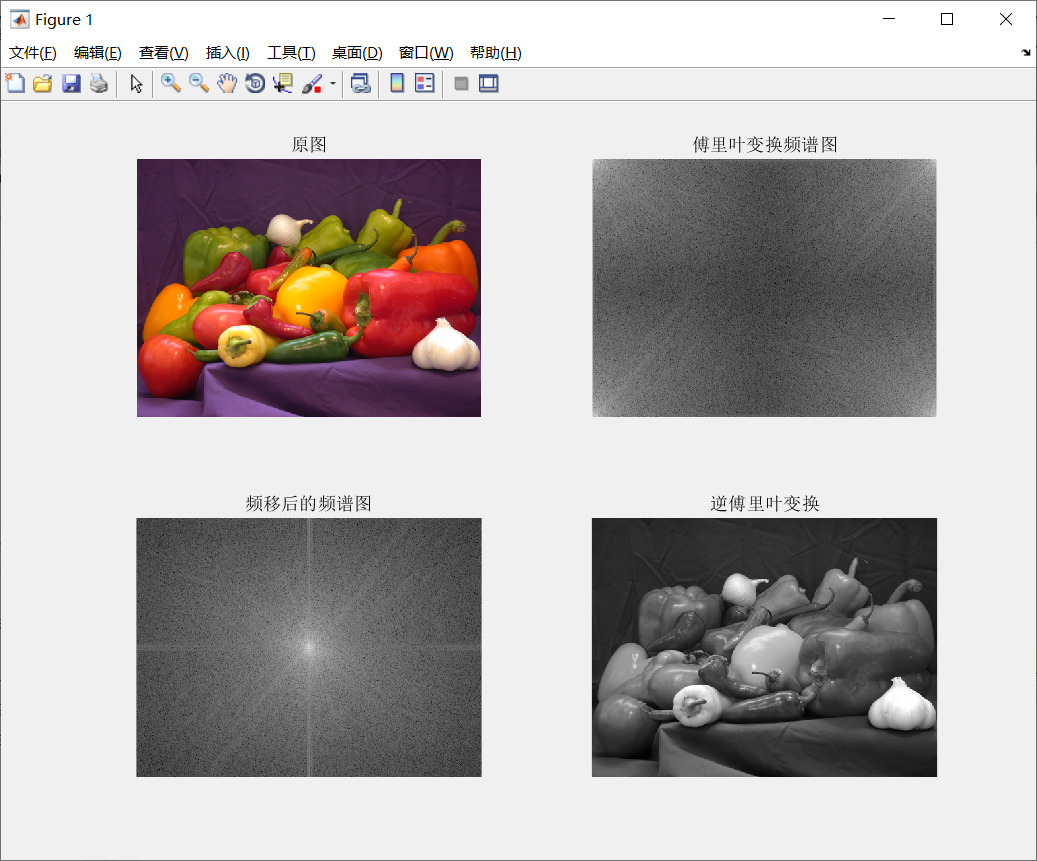图1 MATLAB程序运行后结果
七、实验中遇到的问题及对实验过程的思考
1、关于imshow函数：
使用imshow函数显示图像时要注意图像矩阵类型，当图像是double类型时要使用imshow（I,[]）来根据数据矩阵的数值范围自动设置灰度图像显示范围。
详细可参考matlab官网参考文档：显示图像-MATLAB imshow-MathWorks中国
2、关于运行时可能出现的警告：
如果图像进行傅里叶转换后立即用imshow函数显示，则在命令行可能会显示：Warning: Displaying real part of complex input（警告: 显示复数输入项的实部）。这是因为经过傅里叶变换后的图像矩阵大多是复数矩阵，包含实部和虚部。此时如要显示图像则需要先用abs取复数矩阵的模，再进行显示。
取模后图像矩阵的数值一般会很大，直接用imshow函数是无法显示的，此时可以用log函数取其对数，如log(abs(F)+1)，这样就可以对频谱进行缩放。至于为什么用log(F+1)，如下图所示，对于（0，1）之间的x值经过取对数后会变成负值，而log（x+1）则将所有的x值映射到正数范围内。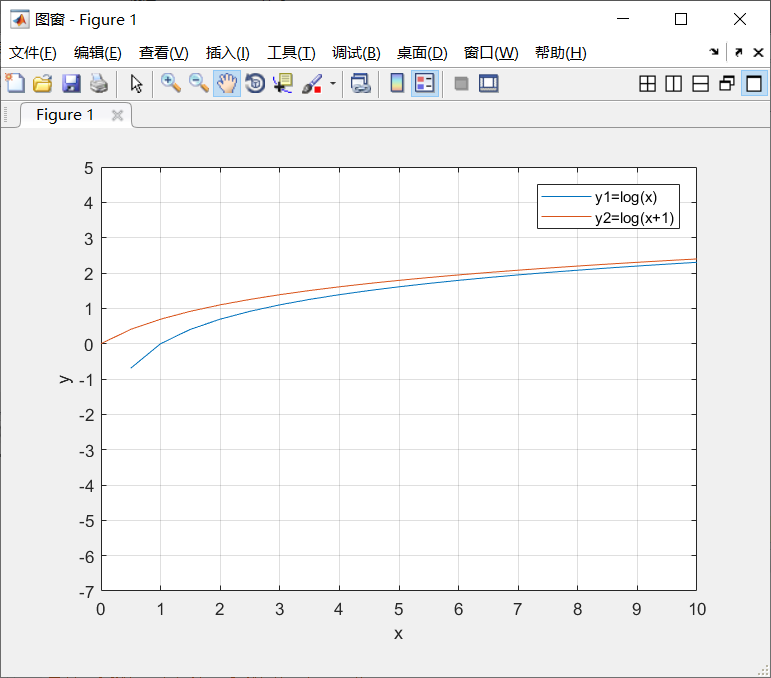图2 log
3、关于开头im2double和rgb2gray函数的使用：
对于RGB真彩图像来说，读入后是以三维矩阵形式存储的，如果此时直接进行傅里叶变换，则频谱图会显示为一片空白或者是密密麻麻的。所以在对RGB图像进行傅里叶变换前要进行类型转换，可以使用im2double将其转换为双精度型（图像运算很少有整型的，所以保守起见不管什么图像都先用这个函数进行转换），或者使用rgb2gray将其转换为灰度图像。
使用不同的函数其变换效果也是不一样的。如使用im2double，进行傅里叶变换后其频谱图呈白色基调，使用rgb2gray其频谱图呈灰色基调。
4、关于最后使用im2uint8转换图像类型：
这一步是为了将逆转换得到的矩阵先转换为灰度图像（mat2gray），再将图像类型转换为uint8。其实这一步的调用与否与开头两个类型转换函数的使用有关，如果在开头就已经将RGB图像转为灰度图像，则此步骤可省去，直接显示经逆转换的图像。如果开头调用im2double，此步省略后可显示出彩色图像。当然此步骤在不同方面发挥着不同作用，取的灰度图像可以为后续操作提供基础。
更多函数信息可参考matlab官网    MATLAB-函数
如有错漏之处敬请指正


展开全文• 运用傅里叶变换对信号进行简单的滤波原理将信号进行傅里叶变换可以信号中有哪些频率成分，将需要滤除的频率成分的幅值置零，然后进行傅里叶逆变换就可以达到滤波的目的。注意点运行FFT进行变换时需要考虑奈奎斯特...运用傅里叶变换对信号进行简单的滤波原理将信号进行傅里叶变换可以信号中有哪些频率成分，将需要滤除的频率成分的幅值置零，然后进行傅里叶逆变换就可以达到滤波的目的。注意点运行FFT进行变换时需要考虑奈奎斯特之后的振幅和相位，进行傅里叶逆变换的时候是取N个点进行变换，而不是取一半。下面以一个实例进行说明信号：x=0.5sin(2pi3t)+cos(2pi10*t)，滤除8Hz-12Hz的信号。dt=0.02;%采样间隔
N=512;%采样点数
t=0:dt:(N-1)*dt;%采样时刻
fs=1/dt;%采样频率，与才采样间隔互为倒数
n=0:1:N-1;
f=(fs/N).*n;%X轴每个点对应的频率
x=0.5*sin(2*pi*3*t)+cos(2*pi*10*t);%信号
figure(1)
plot(t,x)
y=fft(x);%傅里叶变换得到一个复数
Ay=abs(y);%取模
Ayy=Ay*2/N;%转换成实际的幅值
figure(2)
plot(f(1:N/2),Ayy(1:N/2))
f1=8;
f2=15;
yy=zeros(1,length(y));
for m=0:N-1
if(m*(fs/N)>f1&m*(fs/N)&&(fs-f2)&m*(fs/N)<(fs-f1));%将奈奎斯特之后的频率也滤除点掉
yy(m+1)=0;
else
yy(m+1)=y(m+1);
end
end      %将频率为8Hz-12Hz的信号的幅值置0
yyi=abs(yy);
figure(3)
plot(f(1:N/2),yyi(1:N/2))
yi=ifft(yy);
figure(4)
plot(t,real(yi))结果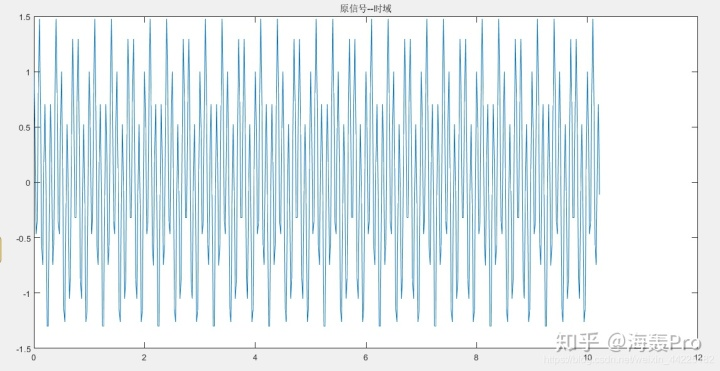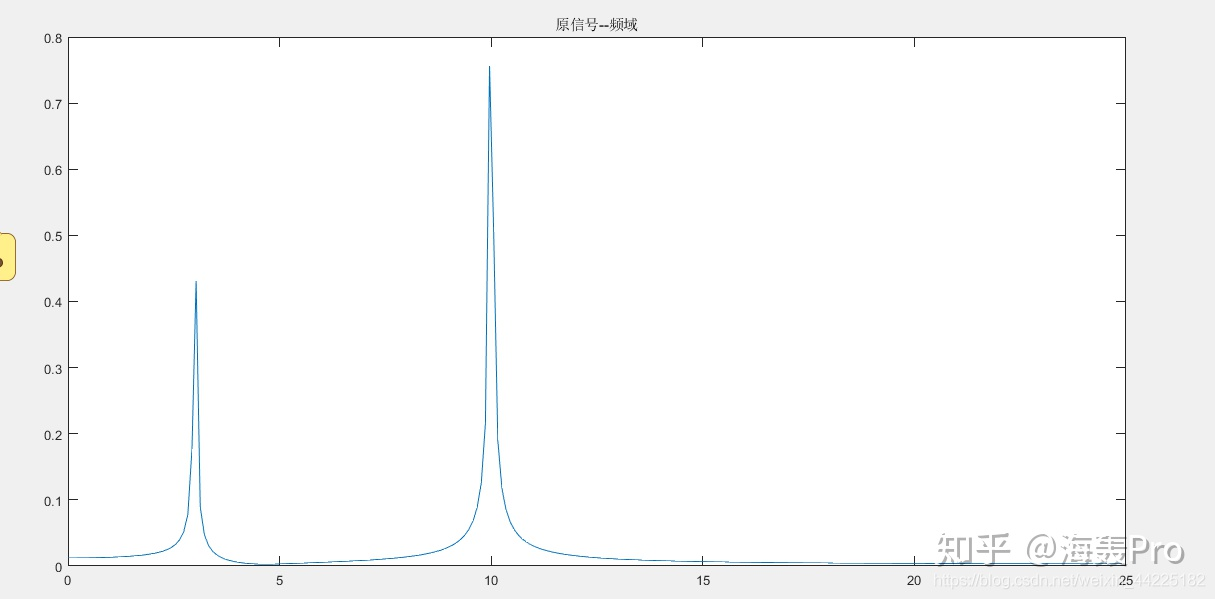滤波后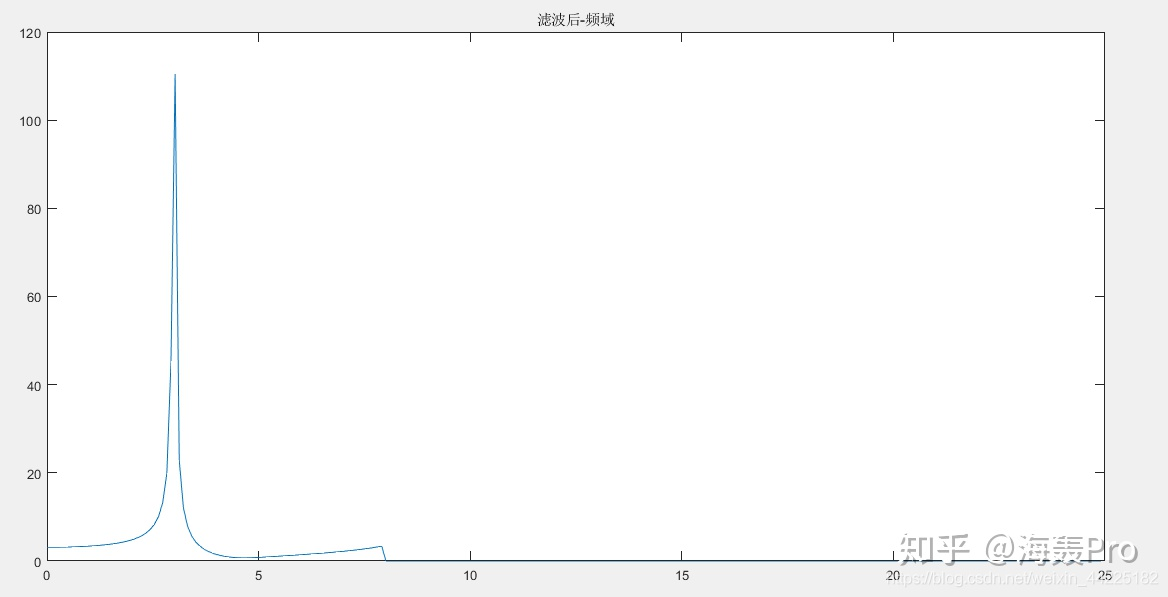展开全文• 原创开发，使用Matlab进行离散傅里叶变换DFT(快速傅里叶变换FFT)进行频谱分析、使用Matlab进行利用离散傅里叶逆变换iDFT从频谱恢复时域信号，内含自定义Matlab函数、丰富的演示实例和详细的说明文档，简单易用。
• 实例分析 2.1离散序列傅里叶变换MATLAB实现 例2.1 已知求其离散时间傅里叶变换并讨论其共轭对称性 根据离散序列傅里叶变换公式将下列指令编辑到 exe2dtft.m 文件中其中[-2,2]并以 % exe2dtft.m 序列的离散时间...
• 用excel如何作快速傅里叶变换？具体实例如下：1.对于时间序列，可以展开成傅立叶级数，进行频谱分析。对于时间序列xt其傅立叶级数展开式为展开成傅立叶级数：由图可见，图形完全对称，通常只取左半部分。频率强度...
用excel如何作快速傅里叶变换？具体实例如下：1.对于时间序列，可以展开成傅立叶级数，进行频谱分析。对于时间序列xt其傅立叶级数展开式为展开成傅立叶级数：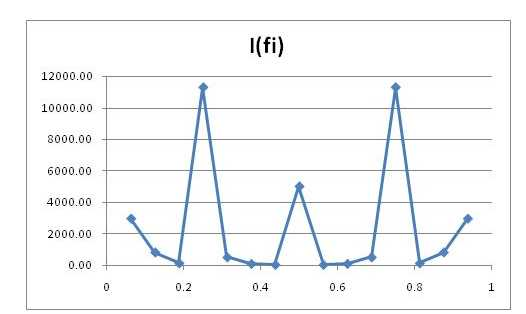由图可见，图形完全对称，通常只取左半部分。频率强度最大的所对应的频率为0.25,其倒数为4,即周期为4.如何对一组数据进行快速傅里叶变换那是因为FFT的结果数值之间差异很大,所以在图上显示不出来,可以只显示部分区间的数值,就是限定坐标值范围。可否用excel做出傅里叶变换用MATLAB 实现傅里叶变换:用户任意输入一个函数，然后，输出函数的傅里叶变换函数，然后输出振幅频率 。x=sin(2*pi*t); %任意输入一个函数。y=fft(x); %傅里叶变换函数。plot(abs(y)); %振幅频率。函数(function)表示每个输入值对应唯一输出值的一种对应关系。这种关系使一个集合里的每一个元素对应到另一个(可能相同的)集合里的唯一元素。函数f中对应输入值的输出值x的标准符号为f(x)。包含某个函数所有的输入值的集合被称作这个函数的定义域，包含所有的输出值的集合被称作值域。若先定义映射的概念，可以简单定义函数为，定义在非空数集之间的映射称为函数。傅里叶变换能将满足一定条件的某个函数表示成三角函数(正弦和/或余弦函数)或者它们的积分的线性组合。在不同的研究领域，傅里叶变换具有多种不同的变体形式，如连续傅里叶变换和离散傅里叶变换。excel中如何进行傅里叶变换傅立叶变换分为四种类别：1、非周期性连续信号傅立叶变换(Fourier Transform, FT)。2、周期性连续信号傅立叶级数(Fourier Series, FS)。3、非周期性离散信号离散时域傅立叶变换(Discrete Time Fourier Transform, DTFT)。4、周期性离散信号离散傅立叶变换(Discrete Fourier Transform, DFT)。Excel的傅立叶分析是快速傅里叶变换(Fast Fourier Transform)及其逆变换。快速傅里叶变换是利用计算机计算离散傅里叶变换(DFT)的高效、快速计算方法的统称。快速傅里叶变换有广泛的应用：数字信号处理、计算大整数乘法、求解偏微分方程、用于判断时间序列周期性。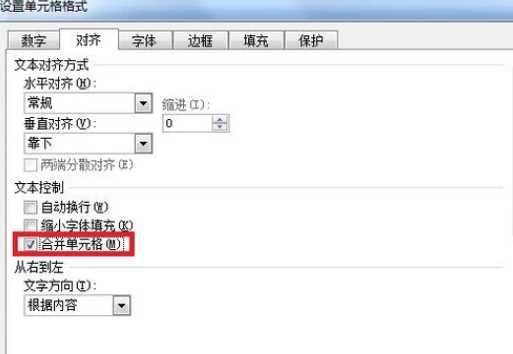扩展资料：傅里叶变换的应用：傅里叶变换是线性算子，若赋予适当的范数，它还是酉算子。傅里叶变换的逆变换容易求出，而且形式与正变换非常类似。正弦基函数是微分运算的本征函数，从而使得线性微分方程的求解可以转化为常系数的代数方程的求解.在线性时不变的物理系统内，频率是个不变的性质，从而系统对于复杂激励的响应可以通过组合其对不同频率正弦信号的响应来获取。著名的卷积定理指出：傅里叶变换可以化复杂的卷积运算为简单的乘积运算，从而提供了计算卷积的一种简单手段。离散形式的傅里叶变换可以利用数字计算机快速的算出(其算法称为快速傅里叶变换算法(FFT))。f(t)是t的周期函数，如果t满足狄里赫莱条件：在一个以2T为周期内f(X)连续或只有有限个第一类间断点，附f(x)单调或可划分成有限个单调区间。则F(x)以2T为周期的傅里叶级数收敛，和函数S(x)也是以2T为周期的周期函数，且在这些间断点上，函数是有限值；在一个周期内具有有限个极值点；绝对可积。如何对一组数据进行快速傅里叶变换那是因为FFT的结果数值之间差异很大,所以在图上显示不出来,可以只显示部分区间的数值,就是限定坐标值范围。快速傅里叶变换是怎么做的用matlab进行傅里叶变换很简单的，直接把你的时间序列数据输进去，然后调用fft函数命令就得到结果了。用matlab比自己写程序方便多了扩展就是说matlab直接就有这个功能？补充对，你只要把数据读进去，然后直接调用fft函数就行了，很简单的如何用matlab对Excel中的数据做傅里叶变换？excel里，excel加载项，分析工具库勾选，里面就有 傅里叶分析，直接用就行了。matlab要调用，最好把excel文件另存为csv文件吧，方便解析。Excel中傅里叶变换求教这里有答案https://zhidao.baidu.com/question/420899476.htmlEXCEL 傅立叶变换没有看到频率的变化范围要取0-1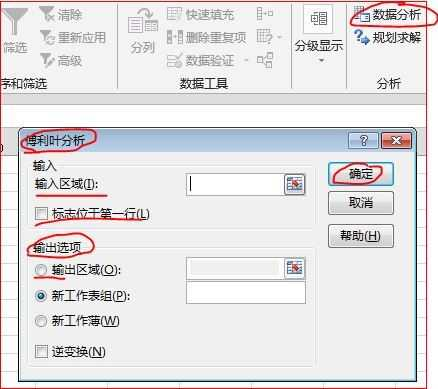扩展谢谢您的回答！您说的这些内容我已经会了。请问您能将时程曲线变换成频谱曲线吗？比方：我有一个txt文件，其中存放了时间和相应的位移，如何在EXCEL中作傅里叶变换成频谱曲线？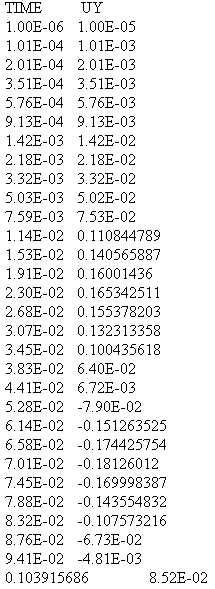补充感谢您的提问，让我开始关注这个分析工具。没有相关的经验，无法提供相应帮助，请关注其他大侠的提示！
展开全文• 离散傅里叶变换（DTFT） MATLAB实例 w = [0:1:500]*pi/500; X= exp(1i*w) ./ (exp(1i*w) - 0.5*ones(1,501)); %ones : Create array of all ones magX= abs(X); angX = angle(X); realX = ...
离散傅里叶变换（DTFT） MATLAB实例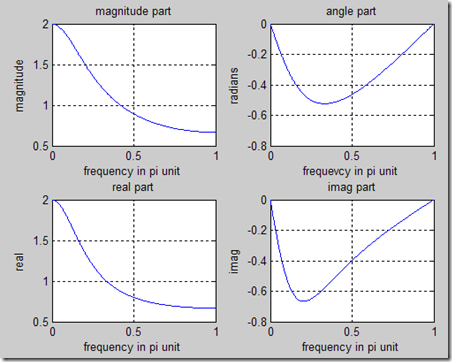w = [0:1:500]*pi/500;
X= exp(1i*w) ./ (exp(1i*w) - 0.5*ones(1,501));        %ones : Create array of all ones

magX= abs(X);
angX = angle(X);
realX = real(X);
imagX = imag(X);
subplot(2,2,1); plot(w/pi, magX);grid
xlabel('frequency in pi unit' );
title ('magnitude part');
ylabel('magnitude');
subplot(2,2,2);plot(w/pi,angX);grid                     %subplot 为图表定位 2X2图表中第2张
xlabel('frequevcy in pi unit');                                 %X轴坐标
title('angle part');
subplot(2,2,3);plot(w/pi, realX );grid                      %grid为图标加网格线
xlabel('frequency in pi unit' );
title('real part');
ylabel('real');
subplot(2,2,4);plot(w/pi, imagX);grid
xlabel('frequency in pi unit');
title('imag part');
ylabel('imag');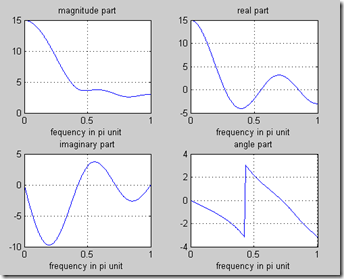n = -1:3;
x = 1:5;
w= (0:1:500)*pi/500;
X= x* exp(-j).^(n'*w);                                          %n'  ：矩阵n的转制
realX = real(X);
imagX = imag(X);
angX = angle(X);
magX = abs(X);
subplot(2,2,1);plot(w/pi,magX);grid
xlabel('fequency in pi unit');
title('magnitude part');
subplot(2,2,2);plot(w/pi,realX);grid
xlabel('frequency in pi unit');
title('real part');
subplot(2,2,3);plot(w/pi,imagX);grid
xlabel('frequency in pi unit');
title( 'imaginary part');
subplot(2,2,4);plot(w/pi, angX);grid
xlabel('frequency in pi unit');
title('angle part');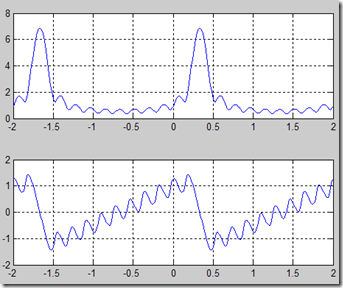%求出某一f上   所有时间上所有值
n= 0:10;%确定n的范围
w= (-200:1:200)*pi/100;%确定要观察的频率范围
x = (0.9*exp(1i*pi/3)).^n;%确定时间轴上的函数值
X = x*(exp(-1i)).^(n'*w);%转换到频率轴上
magX = abs(X);
angX = angle(X);
subplot(2,1,1);
plot(w/pi,magX);grid
subplot(2,1,2);
plot(w/pi, angX);grid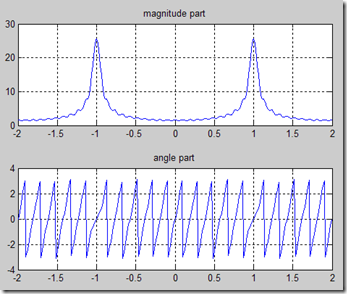%axis 轴        axis()     axis([xmin xmax ymin ymax])
%当输入函数为实数时     观察幅值（偶）与相位（奇）的对称性
n = -10:10 ;
w = (-200:1:200)*pi/100;
x = (-0.9).^n;
X = x * (exp(-1i).^(n'*w));
magX = abs(X);
angX = angle(X);
subplot(2,1,1);
plot(w/pi,magX);grid
axis([-2,2,0,30]);
title('magnitude part');
subplot(2,1,2);
plot(w/pi, angX);grid
title('angle part')


展开全文• 文章目录图像傅里叶变换MATLAB实现图像的二维离散傅里叶变换傅里叶变换后平移变亮后进行傅里叶变换旋转后进行傅里叶变换添加高斯噪声傅里叶反变换 图像的二维离散傅里叶变换 图像的二维离散傅立叶变换，代码如下 %...计算机视觉 图像识别
• 运用傅里叶变换对信号进行简单的滤波原理将信号进行傅里叶变换可以信号中有哪些频率成分，将需要滤除的频率成分的幅值置零，然后进行傅里叶逆变换就可以达到滤波的目的。注意点运行FFT进行变换时需要考虑奈奎斯特...
• 傅里叶分析包括：傅里叶级数（周期信号）和傅里叶变换（非周期函数） 傅里叶变换要求满足狄利克雷条件和（﹣∞，+∞）上绝对可积，正弦函数、单位越阶函数和线性函数等一些...Matlab对图像进行傅里叶变换实例 ...
• Matlab使用杂谈3-Fourier函数实现傅里叶变换傅里叶变换Matlab中的Fourier函数Fourier使用实例普通用法参数变换向量输入傅里叶变换无结果傅里叶逆变换 傅里叶变换 傅里叶展开式(Fourier expansion)是指用三角级数表示...
• MATLAB如何实现傅里叶变换FFT？有何物理意义？ 分步阅读 为什么要进行傅立叶变换，究竟有何意义？如何用MATLAB实现快速傅立叶变换？本文从 FFT 的由来开始讲起，然后在 MATLAB 中实现了 FFT 的计算，...
• 运用傅里叶变换对信号进行简单的滤波 原理 将信号进行傅里叶变换可以信号中有哪些频率成分，将需要滤除的频率成分的幅值置零，然后进行傅里叶逆变换就可以达到滤波的目的。 注意点 运行FFT进行变换时需要考虑...
• 运用傅里叶变换对信号进行简单的滤波原理将信号进行傅里叶变换可以信号中有哪些频率成分，将需要滤除的频率成分的幅值置零，然后进行傅里叶逆变换就可以达到滤波的目的。注意点运行FFT进行变换时需要考虑奈奎斯特...
• 作者简介王雪飞 河北石家庄人...离散傅立叶变换 的一个重要应用就是和作为原模拟信号的频谱近似为说明与原信号频谱的关 系我们设计以下教学实例 例产生一个秒长的包含两个频率分别为 和的连续信号 分析其抽样前后的频谱
• % %图像灰度变换 % f = imread('E:\2013第一学期课程\媒体计算\实验一\Img\Fig0303(a)(breast).tif');% g1 = imadjust(f, [0 1], [1 0]);% g2 = imadjust(f, [0.5 0.75], [0 1]);%...
• clear;FWHM=50e-12;%高斯信号FWHM宽度，为50pstime_window=100*FWHM;%高斯信号的采样窗口宽度，该值决定了傅里叶变换后的频率分辨率Ns=2048;%采样点dt=time_window/(Ns-1);%采样时间间隔t=0:...
• 从时域到频域这里以快速傅里叶变换(FFT)为对象举实例进行解释，并附上全部MATLAB代码。另外，说明一下，用MATLAB做FFT并不要求数据点个数必须为以2为基数的整数次方。之所以很多资料上说控制数据点数为以2为基数的...
• 1.傅里叶变换  傅里叶变换有连续和离散的两种方式，计算机中采用离散的方式，便于计算。一维的离散傅里叶处理单一变量的问题，如声音，二维可以...3.1 离散傅里叶变换matlab实现，左边为50*50的原图，右边为其频谱图
• 以下是我学习傅里叶变换过程中参考的一些书籍、网站等，记录下来，方便以后...(精心整理)图像的傅里叶变换（有例题解释、图像实例介绍和matlab代码） 二维DFT具体实现算法 官方文档：离散傅立叶变换（有opencv实现代图像处理 算法 数学
• 上一篇研究了Matlab如何进行离散傅里叶变换DFT(快速傅里叶变换FFT)进行频谱分析。工程上我们还会遇到这样的问题：获取了信号的频谱，希望从信号的频谱来恢复时域信号。这一篇来研究如何进行利用离散傅里叶逆变换...simulink idft 信号处理 数字信号处理
• 列举了可自行操作的计算机代码及MATLAB实例；介绍了基本理论如何应用于光学、天线、干涉测量、统计、噪声、热学及半导体传播等领域；提供了大量的参考数据表。 本书不仅适合电类专业，同样适合机电信号、物探信号、...
• 从时域到频域这里以快速傅里叶变换(FFT)为对象举实例进行解释，并附上全部MATLAB代码。另外，说明一下，用MATLAB做FFT并不要求数据点个数必须为以2为基数的整数次方。之所以很多资料上说控制数据点数为以2为基数的...
• 从时域到频域这里以快速傅里叶变换(FFT)为对象举实例进行解释，并附上全部MATLAB代码。另外，说明一下，用MATLAB做FFT并不要求数据点个数必须为以2为基数的整数次方。之所以很多资料上说控制数据点数为以2为基数的...
• 连续时间信号的频域分析及matlab实现 离散时间系统的频域分析及matlab实现 傅里叶变换 零极点分析 全部附有编译通过的代码
• matlab、傅里叶、傅里叶变换、FFT、DFT、IFFT、MATLAB中的FFT入门实例教程# matlab傅里叶变换实例matlab 订阅